# The Unit Circle

 [ LiB ]

Circles are found everywhere in the world around us, but to the trigonometry student, few are more important than the unit circle. This is a special case of a circle that has its center on the origin of a Cartesian plane. The unit circle's radius is exactly 1 unit long. This circle is used to define the trigonometric functions that you'll learn later. First, take a look at Figure 11.1, which shows what a unit circle looks like.

##### Figure 11.1. Presenting the unit circle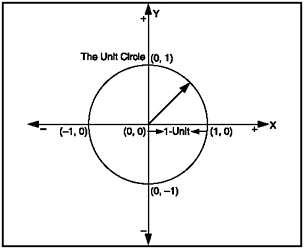A Cartesian plane is a graph of a plane that contains two major axes. Usually they are labeled X and Y and where they intersect is the origin, or (0, 0). If you take a look at Figure 11.2, you can see that the two axes are perpendicular to each other and that this plane contains four quadrants. Moving counter-clockwise, they are Quadrant I, Quadrant II, Quadrant III, and Quadrant IV. The computer screen is like the first quadrant but with an inverted Y axis. In other words, the screen's Y axis gets larger as you move down the screenunlike the Y axis on a Cartesian plane.

##### Figure 11.2. The Cartesian plane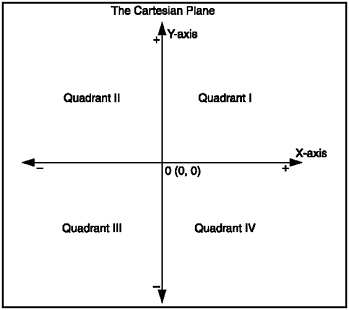NOTE

TIP

If you wanted to graph this circle, the geography way, you could use the equation x ^2 + y ^2 = 1 to solve for each point on the circle.

Pretty soon we're going to go over trigonometric functions and their graphs. For the purposes of this demo, I briefly introduce sine and cosine but don't worry about them too much so that you can focus on how the program creates the circle you will see when you run the program. Sine and cosine can be used to solve for the x and y points around the circumference of a circle, allowing you to draw a circle at any scale. And guess what? This is exactly what I demonstrate in the following listingalso see Figure 11.3.

##### Figure 11.3. Drawing a circle with trigonometry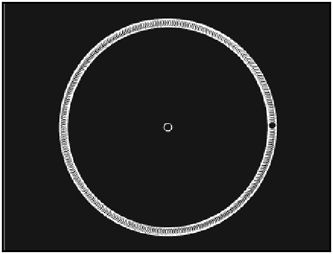Feel free to look into the file GDA_PROG11.1.fla on the CD. You'll see how I set up the file so that I can produce the circle, using trig, which I wanted to produce. The following listing was written up in the "Circle" Movie Clip. In the file, you can double-click on the blue rectangle and look into the following actions in its timeline.

` // Game Development with ActionScript // By Lewis Moronta (c) 2003 // This program uses trig functions // to draw a circle in ActionScript. for (var i = 0; i < 360; i++) {   radians = Math.PI/180 * i;   // Solve for y   var y = Math.sin(radians);   // Solve for x   var x = Math.cos(radians);   // Scale the results for display   x *= 100;   y *= 100;   // Plot the dot =D   plot_dot(x, y, i); } function plot_dot(x, y, dotNum) {   // Create a dot instance   attachMovie("dot", "dot"+dotNum, dotNum);   // Assign their positions...   this["dot"+dotNum]._x = x;   this["dot"+dotNum]._y = y; } `

The first thing I did when planning this demo was to decide where my origin would be. Then I decided how I was going to plot the points. I decided to use a small Movie Clip just big enough to represent a dot.

` function plot_dot(x, y, dotNum) {   // Create a dot instance   attachMovie("dot", "dot"+dotNum, dotNum);     // Assign their positions...   this["dot"+dotNum]._x = x;   this["dot"+dotNum]._y = y; } `

As you can see, this function is a generic function that creates an instance of the "dot" Movie Clip and assigns it to a new position. How does it do this? Well to start off, it has three parameters. One passes the x value, the other passes the y value, and the last passes the instance number that is used in creating the instance (in attachMovie ).

The clip that is being created is referenced dynamicallyyou know how to do that already:

` this["dot"+dotNum]._x = x; this["dot"+dotNum]._y = y; `

The "dot" string is added to dotNum to create a string that represents the instance name this string is then passed on to the this keyword that resolves the Movie Clip instance. This then allows you to use the Movie Clip's methods and properties. I referenced the position properties in this case in order to place the Movie Clip in its new location.

The main code block loops 360 timesone iteration for every degree in a circle. It then uses the Math.sin and Math.cos functions to resolve the x and y values around the circumference. It then scales the coordinate points up to make the circle big enough to be viewed . Right after the positions are resolved, all of these values are passed on to the plot_dot function to actually draw something on the stage.

` for (var i = 0; i < 360; i++) {   radians = Math.PI/180 * i;   // Solve for y   var y = Math.sin(radians);   // Solve for x   var x = Math.cos(radians);   // Scale the results for display   x *= 100;   y *= 100;   // Plot the dot =D   plot_dot(x, y, i); } `

NOTE

NOTE

I scaled the x and y values because sine and cosine only return values from 0 to 1. If the circle were to be plotted that way, you wouldn't notice the circle on the stage because the pixels would be so tight.

So now that you've seen some trigonometry in action,take a look at Figure 11.4 for an explanation of what Math.sin and Math.cos are doing.

##### Figure 11.4. The values retuned by Math.sin and Math.cos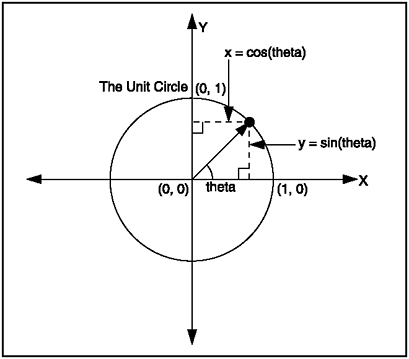As you can see from Figure 11.4, Math.cos returns the x value of the point that lies on the circle. Math.sin returns the y value of where the point is on the circle. By plugging in different values, you can get all the points on the unit circle. Where do you get these values?

 [ LiB ]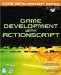Game Development with ActionScript
ISBN: 1592001106
EAN: 2147483647
Year: 2004
Pages: 162
Authors: Lewis Moronta